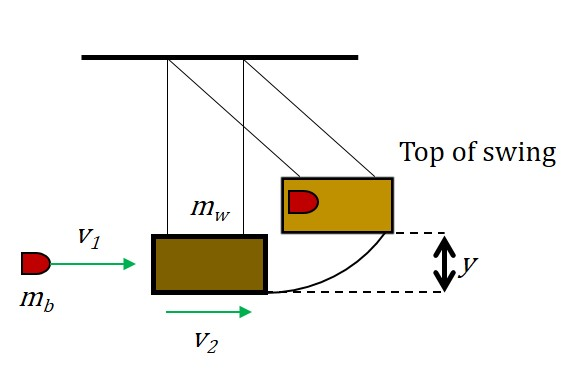# Ballistic Pendulum Puzzle

A ballistic pendulum, as shown, is a system which can be used to measure the speed of a projectile.If $m_{b}=10.0 \text{ g}$ (the mass of the bullet),
$m_{w}=4.00\text{ kg}$ (the mass of the weight),
and $y=4.00\text{ cm}$ (the maximum height of block's swing),
find $v_{1}$, the initial velocity of the bullet in meters per second.

Details and Assumptions

• Take $g$ as $9.81 \text{ms}^{-2}$.
• Give your answer to 3 significant figures.
×

Problem Loading...

Note Loading...

Set Loading...